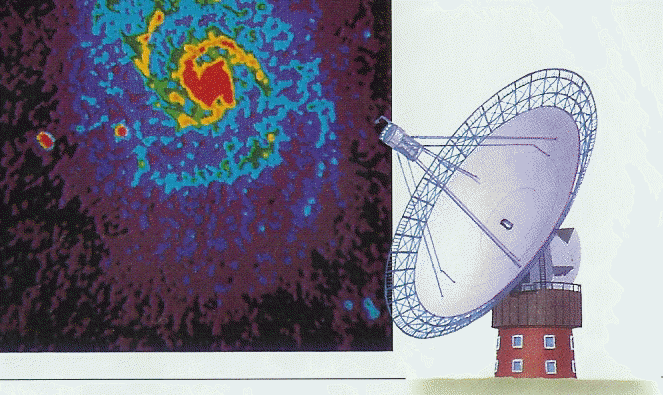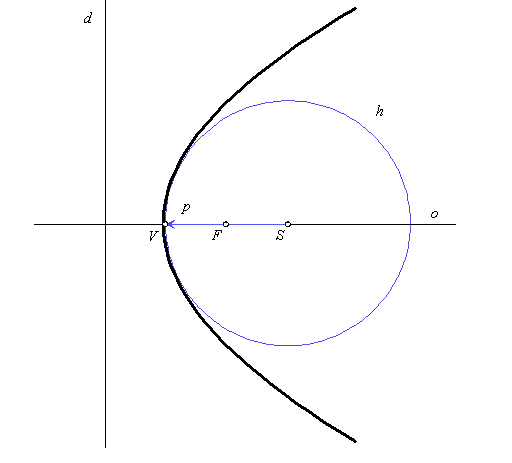# Osculating circle of parabola

In practise, it is very difficult to manufacture large parabolic mirrors, which are used in telescopes.Figure 1: Solar radiotelescope

It is often necessary to make do with mirrors whose cross section is a portion of a circle approximating the appropriate parabola.

It can be shown that the circle that "best approximates" the parabola near its vertex V has its centre S located on the axis o of parabola twice as far from the vertex V as the focus F of the parabola and so it has the radius $p=2|\overline{VF}|=|\overline{dF}|$ equal to the parabola parameter.

This circle $h=\left(S,\phantom{\rule{2mm}{2mm}}p\right)$ is called the osculating circle of a parabola in its vertex V.

Osculating circle and parabola have common tangent perpendicular to the parabola axis, and the same first curvature in their common point - parabola vertex V, see figure.Figure 2: Parabola with osculating circle